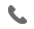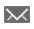•+44 7459 302492info@uplatz.com
• Register
Job Meter = High

### Optimization Concepts for Data Science

30 Hours
Online Instructor-led Training
USD 1399 (USD 2800)
Save 50% Offer ends on 31-Dec-2023318 Learners

This course focuses on linear, nonlinear, and efficiency optimization concepts. You learn how to formulate optimization problems and how to make their formulations efficient by using index sets and arrays. The demonstrations in the course include examples of data envelopment analysis and portfolio optimization. The OPTMODEL procedure is used to solve optimization problems that reinforce concepts introduced in the course.

The e-learning format of this course includes Virtual Lab time to practice.

Learn how to
• Identify and formulate appropriate approaches to solving various linear and nonlinear optimization problems.
• Create optimization models commonly used in industry.
• Formulate and solve a data envelopment analysis.
• Solve optimization problems using the OPTMODEL procedure in SAS.
--------------------------------------------------------------------------
Target Audience

Those who want to develop the advanced knowledge and skills necessary to work as a data scientist, especially those with a strong background in applied mathematics
--------------------------------------------------------------------------

# Optimization Concepts for Data Science

Course Details & Curriculum
Introduction to Mathematical Optimization
• Introduction.
• A simple example.
• The OPTMODEL procedure.
Linear Programming Problems: Basic Ideas
• Introduction to linear programming.
• Formulating and solving linear programming problems using the OPTMODEL procedure.
• Using index sets and arrays in the OPTMODEL procedure.
• Dual values and reduced costs in the simplex method (self-study).
• Applied data envelopment analysis.
• Reading SAS data sets (self-study).
Nonlinear Programming Problems
• Introduction to nonlinear programming.
• Solving nonlinear programming problems using the OPTMODEL procedure.
--------------------------------------------------------------------------